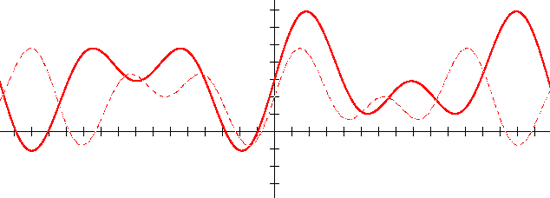Home > General Resources > Recent Talks > Dynamic Algebra

# Dynamic Algebra Software: Bringing Functions Alive with Sketchpad

2006 NCTM Annual Meeting, Session 836.1

Scott Steketee
Key Curriculum Press, Emeryville, CaliforniaThe sketches and the student sheets for many of the activities used in this presentation are linked to the right.

#### Dynamic Investigation of Functions

The behavior of functions is a fundamental unifying concept in algebra. Because variables change, it’s difficult to use static media—like textbook pages and chalk boards—to represent the interplay between the independent and dependent variables of a function. Similarly, static media aren’t suited to showing families of functions or the way in which one function can be transformed into another. Dynamic mathematics software like The Geometer’s Sketchpad allows variables to vary, makes possible a variety of representations of functions, and allows functions to be manipulated and transformed.

In this session we look at a number of activities in which the power of dynamic mathematics software gives students the opportunity to vary the variables, generate families of functions, and transform functions.

These activities are designed for students to use in a computer lab (preferably with two students at each computer). Each activity includes student activity sheets (to guide students through the activity), activity notes (to provide teachers with guidance, commentary, and answers to the activity questions), and presenter notes (to facilitate the use of the activity as a whole-class presentation).

#### From Dynagraphs to Cartesian Graphs

In this activity students make connections between symbolic and dynagraph representations of functions by matching dynagraphs with equations, and then generate a Cartesian representation by geometrically manipulating a dynagraph.

#### Graphing Inequalities in Two Variables

In this activity students explore the graphs of inequalities by dragging a point on the Cartesian plane and observing where the inequality is true and where it is false. Students investigate the relationship between an inequality and the corresponding equation, and compare the graphs of different (but related) inequalities.

#### Parabolas in Factored Form

In this activity students plot the graph of a quadratic function in factored form, investigate the relationship between this form and its graph, and use their observations to create functions from various descriptions of their graphs.

#### Stretching and Shrinking Functions

In this activity students stretch and shrink function graphs vertically and horizontally by multiplying the function value and dividing the function argument by constants.

#### Unit Circle Functions

In this activity students use a unit circle to define the trigonometric functions. They construct a point on the unit circle, measure the coordinates of the point, and graph the coordinates as a function of the arc angle from the positive x-axis to the point, producing plots of the sine and cosine functions. They also plot the slope of the line from the origin through the point, producing a plot of the tangent function.

#### Box and Whisker Plots

In this activity students manipulate data points and observe the effect of their changes on a box and whisker plot. In the process they gain an intuitive understanding of the box and whisker representation of data distribution.

#### Fitting Functions to Data

In this activity students transform functions to fit data, using a least-squares calculation to judge how good the fit is. They use several different parent functions, depending on the shape of the data. (The presentation sketch is not yet available for this activity.)

 The term dynagraph was coined by Paul Goldenberg, Philip Lewis, and James O’Keefe in their study “Dynamic Representation and the Development of a Process Understanding of Functions” published by Education Development Center, Inc., and supported in part by a grant from the National Science Foundation.